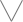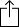C
D
E
F
G
A
B
Chord
Scale
3nps
CAGED
None
Notes
Degrees
Intervals
None

1
2
3
4
5
6
7
1
2
3
4
5
6
7
8
9
10
11
12
13
14
15
16
17
18
19
20
21
22
23
24
E
B
G
D
A
E
B
F
G
G
A
A
B
B
C
D
D
E
E
F
G
G
A
A
B
B
C
D
D
E
E
C
D
D
E
E
F
G
G
A
A
B
B
C
D
D
E
E
F
G
G
A
A
B
B
A
A
B
B
C
D
D
E
E
F
G
G
A
A
B
B
C
D
D
E
E
F
G
G
E
E
F
G
G
A
A
B
B
C
D
D
E
E
F
G
G
A
A
B
B
C
D
D
B
B
C
D
D
E
E
F
G
G
A
A
B
B
C
D
D
E
E
F
G
G
A
A
F
G
G
A
A
B
B
C
D
D
E
E
F
G
G
A
A
B
B
C
D
D
E
E
C
D
D
E
E
F
G
G
A
A
B
B
C
D
D
E
E
F
G
G
A
A
B
B

# D Minor Eleventh for 7 String Guitar - 3nps Chart

A D Minor eleventh chord, also written as Dm11, D-11 or Dmin11 contains the notes D, F, A, C, E and G. The chord is comprised of the intervals m3, M3, m3, M3 and m3.

D
F
A
C
E
Gm3
M3
m3
M3
m3
Play scaleand then